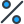# What is the difference? StartFraction x Over x squared + 3 x + 2 EndFraction minus StartFraction 1 Over (x + 2) (x + 1) EndFraction options A)StartFraction x minus 1 Over 6 x + 4 EndFraction B)StartFraction negative 1 Over 4 x + 2 EndFraction C)StartFraction 1 Over x + 2 EndFraction D)StartFraction x minus 1 Over x squared + 3 x + 2 EndFraction

•Subject:

Mathematics
• Author:

silky
• Created:

7 months ago

D

Step-by-step explanation:

x/(x²+3x+2) - 1/[(x+2)(x+1)]

x² + 3x + 2 = x² + 2x + x + 2

= x(x + 2) + (x + 2)

= (x + 2)(x + 1)

= x/[(x+2)(x+1)] - 1/[(x+2)(x+1)]

= (x-1)/[(x+2)(x+1)]

= (x-1)/(x²+3x+2)

The difference of rational polynomial function is \frac{x-1}{x^{2} +3x+2}

Given expression is,

\frac{x}{x^{2} +3x+2}-\frac{1}{(x+2)(x+1)}

(x+2)(x+1)=x^{2} +2x+x+2=x^{2} +3x+2

Given expression can be written as,

\frac{x}{x^{2} +3x+2}-\frac{1}{x^{2} +3x+2} =\frac{x-1}{x^{2} +3x+2}

Answer: D)StartFraction x minus 1 Over x squared + 3 x + 2 EndFraction Explanation: The difference between the two fractions can be found by subtracting the numerators and denominators from each other. The numerator of the first fraction is "x" and the numerator of the second fraction is "1". Therefore, the difference is "x-1". The denominator of the first fraction is "x^2+3x+2" and the denominator of the second fraction is "(x+2)(x+1)". Simplifying, this becomes "x^2+3x+2". Therefore, the difference in the denominator is also "x^2+3x+2". As such, the difference is "StartFraction x minus 1 Over x squared + 3 x + 2 EndFraction".

Don't worry! There are several alternative approaches you can try to resolve your query. Here are some tips to help you find answers in different ways:

1. Reframe your question: Sometimes, the way you phrase your question can limit your search results. Try rephrasing it using different keywords or providing more context to get better results.
2. Utilize social media: Post your question on social media platforms, particularly those focused on professional or specialized topics. Twitter, LinkedIn, and Facebook groups can connect you with individuals who may have relevant expertise or experiences to share.
3. Consult subject matter experts: Reach out to experts in the field related to your question. Many professionals are willing to help and share their knowledge. You can connect with them through email, LinkedIn messages, or by attending relevant conferences or events.
4. Use our website to find your question through the search box above, or you can sign up to ask your question for our big educational community. Our experts will review your question, and you will get a quick and quality answer.
5. Collaborate with others: If your question is related to a specific project or problem, consider collaborating with others who might have complementary skills or knowledge. Teamwork can lead to innovative solutions and shared insights.

Remember, the process of finding answers often involves persistence, creativity, and an open mind. By exploring various resources, reaching out to others, and being proactive in your search, you increase your chances of finding the information you need. Happy quest for knowledge!